# Chapter # 04 : Searching & Types | Binary Tree

### Searching

Computer systems are often used to store large amounts of data from which individual records must be retrieved according to some search criterion. Thus the efficient storage of data to facilitate fast searching is an important issue.

### Sequential Search

Linear search or sequential search is a method for finding a target value within a list. It sequentially checks each element of the list for the target value until a match is found or until all the elements have been searched

Number => 33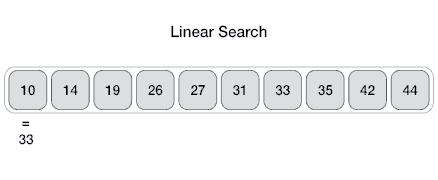Figure 1​​ Step1

Checking on index 0, and value on index 0 is not matched move next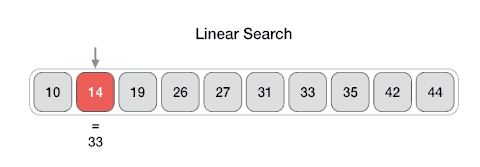Figure 2​​ Step2

Checking on index 1, and value on index 1 is not matched move next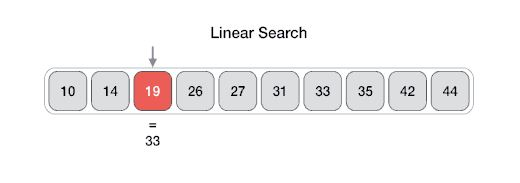Figure 3​​ Step 3

Checking on index 2, and value on index 2 is not matched move next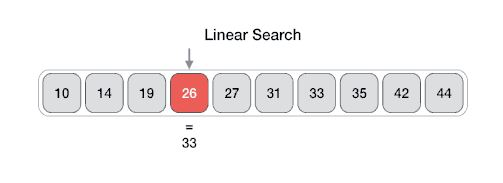Figure 4​​ Step 4

Checking on index 3, and value on index 3 is not matched move next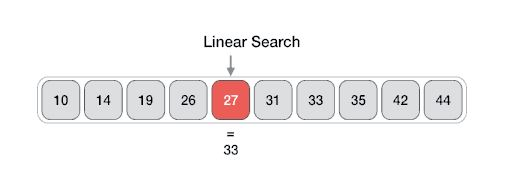Figure 5​​ Step 5

Checking on index 4, and value on index 4 is not matched move next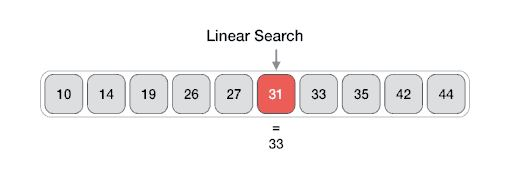Figure 6​​ Step 6

Checking on index 5, and value on index 5 is not matched move next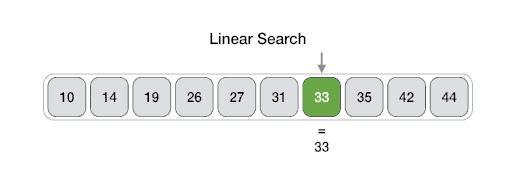Figure 7​​ Step 6

Checking on index 6, and value on index 6 is not matched move next

### Algorithm

Linear Search ( Array A, Value x)

Step 1: Set i to 0// from 0 index

Step 2: if i > n then go to step 7

Step 3: if A[i] = x then go to step 6

Step 4: Set i to i + 1

Step 5: Go to Step 2

Step 6: Print Element x Found at index i and go to step 8

Step 8: Exit

### Pseudocode

Procedure linear_search (list, value)

​​ ​​​​ for each item in the list

​​ ​​ ​​ ​​ ​​​​ if match item == value

​​ ​​ ​​ ​​ ​​ ​​ ​​ ​​​​ return the item's location

​​ ​​ ​​ ​​ ​​​​ end if

​​ ​​​​ end for

end procedure

### Binary Search

For a binary search to work, it is mandatory for the target array to be sorted. We shall learn the process of binary search with a pictorial example. The following is our sorted array and let us assume that we need to search the location of value 31 using binary search.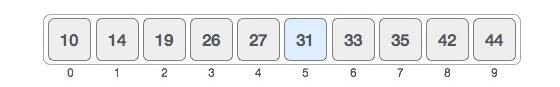First, we shall determine half of the array by using this formula.

mid = low + ((high - low) / 2)

Here it is, 0 + (9 - 0) / 2 = 4 (integer value of 4.5). So, 4 is the mid of the array.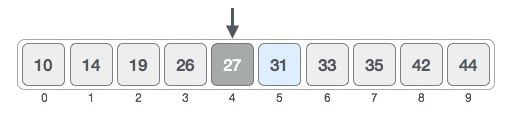Now we compare the value stored at location 4, with the value being searched, i.e. 31. We find that the value at location 4 is 27, which is not a match. As the value is greater than 27 and we have a sorted array, so we also know that the target value must be in the upper portion of the array.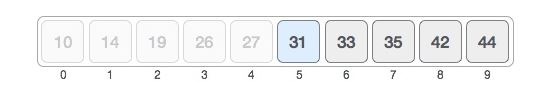We change our low to mid + 1 and find the new mid value again.

low = mid + 1

mid = low + (high - low) / 2

Our new mid is 7 now. We compare the value stored at location 7 with our target value 31.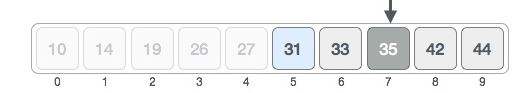The value stored at location 7 is not a match, rather it is more than what we are looking for. So, the value must be in the lower part from this location.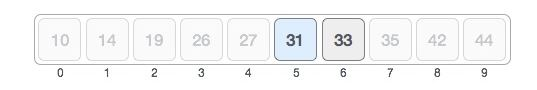Hence, we calculate the mid again. This time it is 5.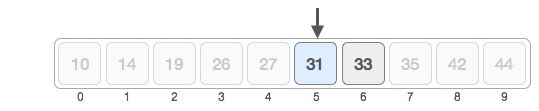We compare the value stored at location 5 with our target value. We find that it is a match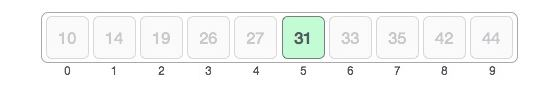We conclude that the target value 31 is stored at location 5.

Binary search halves the searchable items and thus reduces the count of comparisons to be made to very less numbers.

### Pseudocode

Procedure binary_search

​​ ​​​​ A sorted array

​​ ​​​​ n size of array

​​ ​​​​ x value to be searched

​​ ​​​​ Set lowerBound = 1

​​ ​​​​ Set upperBound = n

​​ ​​ ​​ ​​ ​​​​ if upperBound < lowerBound

​​ ​​ ​​ ​​ ​​ ​​ ​​ ​​​​ EXIT: x does not exists.

​​ ​​​​

​​ ​​ ​​ ​​ ​​​​ set midPoint = lowerBound + ( upperBound - lowerBound ) / 2

​​ ​​ ​​ ​​ ​​​​

​​ ​​ ​​ ​​ ​​​​ if A[midPoint] < x

​​ ​​ ​​ ​​ ​​ ​​ ​​ ​​​​ set lowerBound = midPoint + 1

​​ ​​ ​​ ​​ ​​ ​​ ​​ ​​​​

​​ ​​ ​​ ​​ ​​​​ if A[midPoint] > x

​​ ​​ ​​ ​​ ​​ ​​ ​​ ​​​​ set upperBound = midPoint - 1

​​ ​​ ​​ ​​ ​​​​ if A[midPoint] = x

​​ ​​ ​​ ​​ ​​ ​​ ​​ ​​​​ EXIT: x found at location midPoint

​​ ​​​​ end while

​​ ​​​​

end procedure

### Tree

When starting out programming, it is common to understand better the linear data structures than data structures like trees and graphs.

Trees are well-known as a non-linear data structure. They don’t store data in a linear way. They organize data hierarchically.

* Binary tree = tree where each node has at most 2 children nodes

In computer science, a binary tree is a tree data structure in which each node has at the most two children, which are referred to as the left child and the right child

## Terminology used in trees

• Root is the topmost node of the tree

Node at the "top" of a tree - the one from which all operations on the tree commence. The root node may not exist (a NULL tree with no nodes in it) or have 0, 1 or 2 children in a binary tree.

• Edge is the link between two nodes

• Child is a node that has a parent node

• Parent is a node that has an edge to a child node

• Leaf is a node that does not have a child node in the tree

Node at the "bottom" of a tree - farthest from the root. Leaf nodes have no children.

• Height is the length of the longest path to a leaf

Number of nodes which must be traversed from the root to reach a leaf of a tree.

• Depth is the length of the path to its root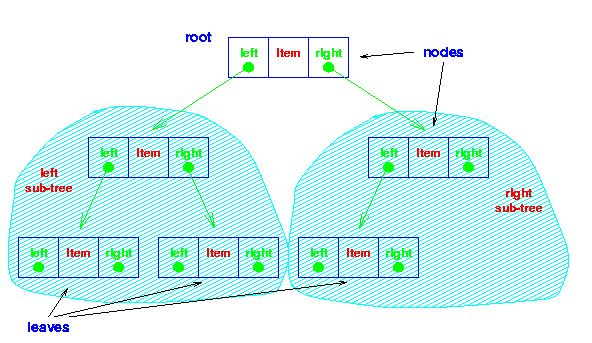Figure 8​​ Example of Tree

### Complete Tree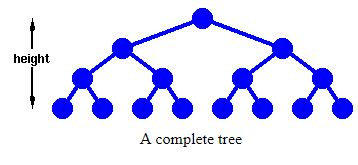Definition:

This forms a complete tree, whose height is defined as the number of links from the root to the deepest leaf. Tree in which each leaf is at the same distance from the root.

First, we need to work out how many nodes, n, we have in such a tree of height, h.

Now,

Total number of Nodes.

n = 1 + 21 + 22 +.... + 2h

From which we have,

n = 2h+1 – 1

Detailed Explanation: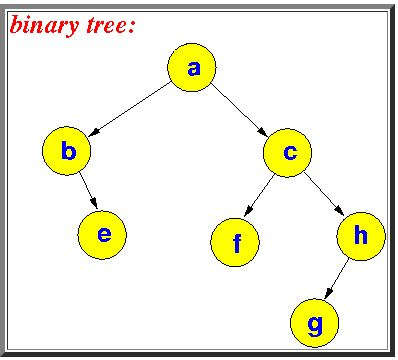Figure 9​​ A tree where each node has at most 2 children nodes

Left and right child

Because each node has at most 2 children nodes, we can label the children distinctly as left and right: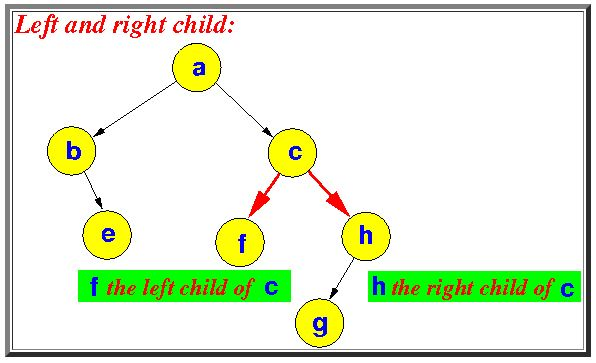Note

• Some nodes (e.g. h) have only a left child node

• Some nodes (e.g. b) have only a right child node

Perfect binary tree

A binary tree where each level contains the maximum number of nodes

I.e., every level is completely full of nodes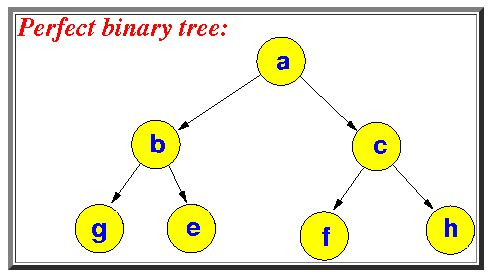Some properties of the perfect binary tree

Depth of Tree

• The number of nodes at depth d in a perfect binary tree = 2d

There is only 1 node (= the root node) at depth 0: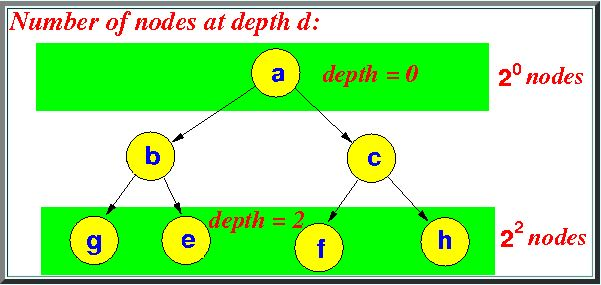Figure 10, in a perfect binary tree, every node has 2 children nodes

So: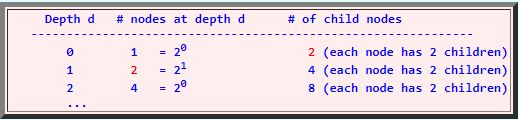Figure 11​​ the number of nodes doubles every time the depth increases by 1!

Therefore:

​​ ​​​​ # Nodes at depth d = 2d

Total Number of Node

The total number of nodes in a perfect binary tree of height h: has 2h+1 − 1 nodes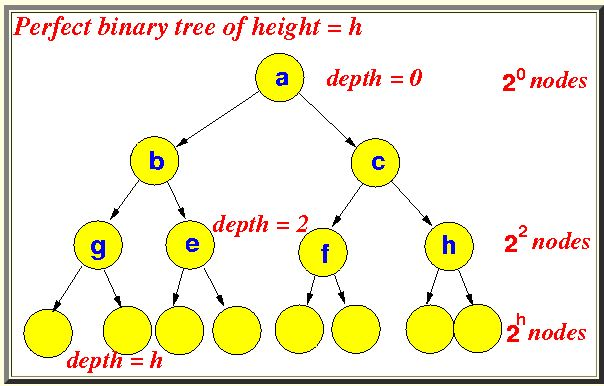Figure 12​​ # nodes = 20 + 21 + ... 2h = 2h+1 − 1

Therefore

Height h=3;

By using Formula 23+1 – 1=24-1;

=16-1

=15 Nodes, all we have the Maximum number of Nodes in tree.

Total Number of Leaf nodes

• All the leaf nodes in a perfect binary tree of height h has a depth equal to h: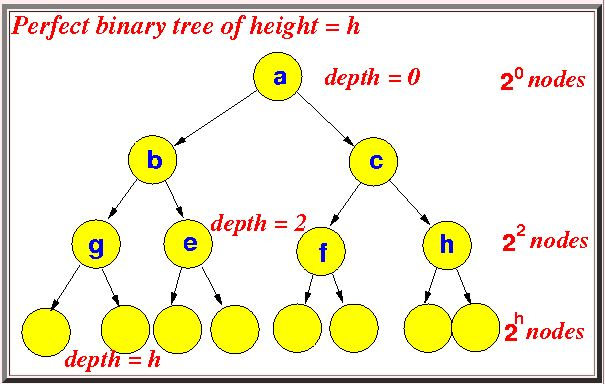Figure 13​​ # nodes at depth h in a perfect binary tree = 2h

Therefore

Number of leaf nodes in a perfect binary tree of height h = 2h

So the Height h =3;

By using Formula 2h=23;

Total number of Leafs=8 Ans

Number of internal nodes

• Number of internal nodes in a perfect binary tree of height h = 2h − 1

# Nodes in a perfect binary tree of height h = 2h+1 − 1  ​​​​

# Leaf nodes in a perfect binary tree of height h = 2h  ​​​​

The other nodes are internal nodes (i.e., with at least 1 child node).

So:

# Internal nodes in a perfect binary tree of

Height h = (2h+1 − 1) − 2h = 2h − 1